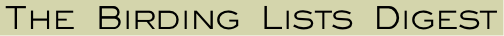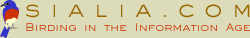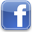Date: 4/22/19 5:58 pmFrom: marvin myers Subject: Re: [ia-bird] Digest for - 19 updates in 16 topics Whoops!!!Sent from my iPhone> On Apr 22, 2019, at 7:37 PM, wrote:> > =3D=3D=3D=3D=3D=3D=3D=3D=3D=3D=3D=3D=3D=3D=3D=3D=3D=3D=3D=3D=3D=3D=3D=3D=3D=> =3D=3D=3D=3D=3D=3D=3D=3D=3D=3D=3D=3D=3D=3D=3D=3D=3D=3D=3D=3D=3D=3D=3D=3D=3D=> =3D=3D=3D=3D=3D=3D=3D=3D=3D=3D=3D=3D=3D=3D=3D=3D=3D=3D=3D=3D=3D=3D=3D=3D=3D=> =3D=3D> Today's topic summary> =3D=3D=3D=3D=3D=3D=3D=3D=3D=3D=3D=3D=3D=3D=3D=3D=3D=3D=3D=3D=3D=3D=3D=3D=3D=> =3D=3D=3D=3D=3D=3D=3D=3D=3D=3D=3D=3D=3D-- You received this message because you are subscribed to the Google Groups "IA-BIRD" group.To unsubscribe from this group and stop receiving emails from it, send an email to ia-bird+To post to this group, send email to Visit this group at https://groups.google.com/group/ia-bird.To view this discussion on the web visit https://groups.google.com/d/msgid/ia-bird/For more options, visit https://groups.google.com/d/optout.Join us on Facebook!